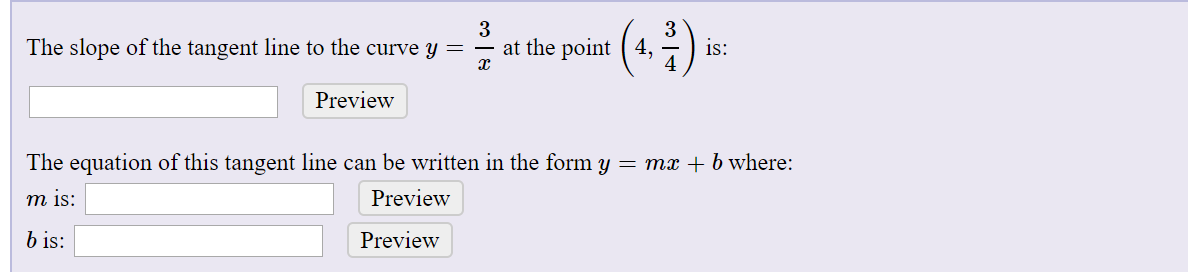# (*.:)-3at the point ( 4,The slope of the tangent line to the curve y =is:4PreviewThe equation of this tangent line can be written in the form y = mx + b where:Previewm is:b is:Preview

Question
1 viewshelp_outlineImage Transcriptionclose(*.:)- 3 at the point ( 4, The slope of the tangent line to the curve y = is: 4 Preview The equation of this tangent line can be written in the form y = mx + b where: Preview m is: b is: Preview fullscreen
check_circle

Step 1

Given,

Step 2

Slope of the tangent is the derivative of the function.

Therefore differentiating the given curve with respect to x, we get

Step 3

Now

...

### Want to see the full answer?

See Solution

#### Want to see this answer and more?

Solutions are written by subject experts who are available 24/7. Questions are typically answered within 1 hour.*

See Solution
*Response times may vary by subject and question.
Tagged in

### Derivative